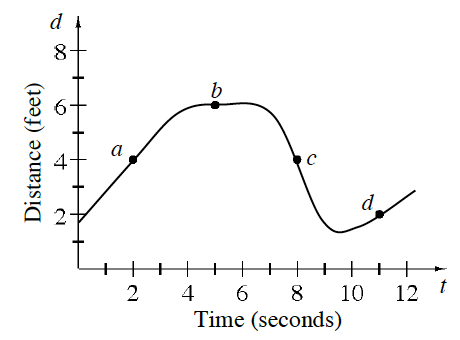### Home > APCALC > Chapter 2 > Lesson 2.2.1 > Problem2-56

2-56.

Zuhaib is anxiously waiting for the results of his calculus test and is pacing back and forth as shown in the graph below.1. At which point $(a, b, c, \text{ or } d)$ is Zuhaib’s speed the greatest? Approximate the rate.

This is a distance/position graph. Notice how Zuhaib is moving forward and backwards as she paces.

Speed is the absolute value of velocity. And velocity is the slope of a distance graph.

At which point is the absolute value of the slope the steepest?

2. At which point is Zuhaib’s velocity the greatest? Approximate the rate.

Velocity is the slope on a distance/position graph.

3. What is the difference between speed and velocity?

Speed is the absolute value of velocity.